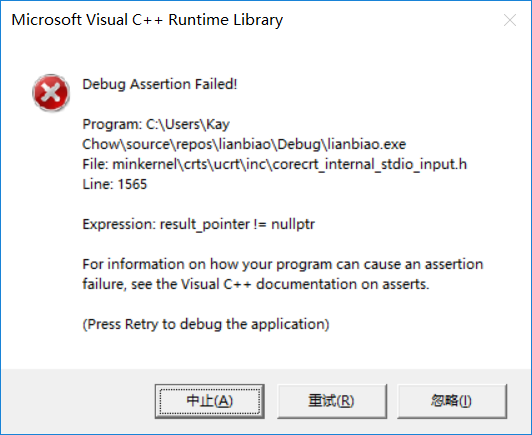#include
#include
#include

#define LEN sizeof(struct student)

struct student *creat();

struct student
{
int num;
float score;
struct student *next;
};

int n;

void main()
{
struct student *stu;

``````stu = creat();
print( stu );

printf("\n\n");
system("pause");
``````

}

struct student *creat()
{
struct student *p1, *p2;

``````p1 = p2 = (struct student *)malloc(LEN);

scanf_s("%d", p1->num,sizeof(p1->num));
scanf_s("%f", p1->score,sizeof(p1->score));

n = 0;

while (p1->num)
{
n++;
if (n == 1)
{
}
else
{
p2->next = p1;
}
p2 = p1;
p1 = (struct student *)malloc(LEN);

scanf_s("%d", p1->num, sizeof(p1->num));
scanf_s("%f", p1->score, sizeof(p1->score));
}
p2->next = NULL;

``````

}

{
struct student *p;
while (p->next)
{
printf("学号： %d 分数： %f\n", p->num, p->score);
p = p->next;
}
}

0x0F86742E (ucrtbased.dll)处(位于 lianbiao.exe 中)引发的异常: 0xC0000005: 写入位置 0xCDCDCDCD 时发生访问冲突。2个回答

``````scanf_s("%d", p1->num, sizeof(p1->num));
``````

``````scanf_s("%d", &p1->num, sizeof(p1->num));
``````

stu = creat();???